NCERT Solutions for Class 12 Chemistry Chapter 3 provides detailed solutions for the questions asked in the textbook. The solutions are provided by subject experts and the students can refer to these to prepare well for the exams. NCERT Solutions are a guide to the students appearing in different boards like MP board, UP board, CBSE, Gujarat board, etc.

Chemistry Class 12 Chapter 3 Electrochemistry is very important from the examination point of view. All the analytical and conceptual details are provided in the NCERT Solutions Class 12 Chapter 3 that will help the students to score well.

 Board CBSE Textbook NCERT Class Class 12 Subject Chemistry Chapter Chapter 3 Chapter Name Electrochemistry Number of Questions Solved 33 Category NCERT Solutions

## NCERT Solutions for Class 12 Chemistry Chapter 3 Electrochemistry

The production of electricity from chemical reactions and the use of electrical energy to bring non-spontaneous chemical transformations is known as electrochemistry. Both the theory and the practical portions are very important in this chapter.

Class 12 Chemistry chapter 3 Electrochemistry explains different types of cells and the differences between the two. Important concepts such as Gibb’s energy and equilibrium constant are also discussed here.

NCERT IN-TEXT QUESTIONS

Question 1.
How would you determine the standard electrode potential of the system ; Mg2+/Mg ?
n order to determine E° value of Mg2+/Mg electrode, an electrochemical cell is set up in which a Mg electrode dipped in 1 M MgSO4 solution acts as one half cell (oxidation half cell) while the standard hydrogen electrode acts as the other half cell (reduction half cell). The deflection of voltmeter placed in the cell circuit is towards the Mg electrode indicating the flow of current. The cell may be represented as :
Mg/Mg2+ (1 M) || H+(l M)/H2(1 atm), Pt
The reading as given by voltmeter gives $${ E }_{ cell }^{ \circ }$$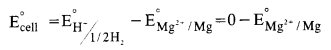The expected value of standard electrode potential (E°) = -2-36 V.

Question 2.
Can you store copper sulphate solution in a zinc pot ?
No, it is not possible. The E° values of the copper and zinc electrodes are as follows :
Zn2+(aq) + 2e → Zn(s) ; E° = – 0·76 V
Cu2+(aq) + 2e → Cu(s) ; E° = + 0·34 V
This shows that zinc is a stronger reducing agent than copper. It will lose electrons to Cu2+ ions and redox reaction will immediately set in.
Zn(s) + Cu2+ (aq) → Zn2+(aq) + Cu(s)
Thus, copper sulphate solution cannot be stored in zinc pot.

Question 3.
Consultthe table of standard electrode potentials and suggest three substances that can oxidise ferrous ions under suitable conditions.
Oxidation of Fe2+ converts it to Fe3+, i.e.,Fe2+ –>Fe3+ +e ; E°ox= – 0.77 V Only those substances can oxidise Fe2+ to Fe3+ which are stronger oxidizing agents and have positive reduction potentials greater than 0.77 V, so that EMF of the cell reaction is positive. This is so for elements lying below Fe3+/Fe2+ in the series ex: Br2, Cl2 and F2.

Question 4.
Calculate the potential of hydrogen electrode in contact with a solution with pH equal to 10.
For hydrogen electrode, H+ + e → 1/2H2
Applying Nernst equation,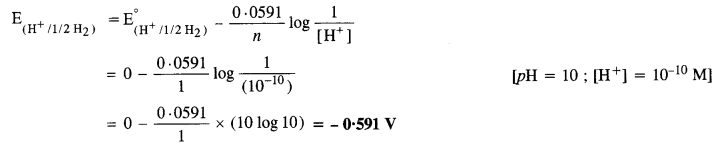Question 5.
Calculate e.m.f. of the cell in which the following reaction takes place
Ni(s) + 2Ag+(0·002M) → Ni2+(0·160M) + 2Ag(s) Given that $${ E }_{ cell }^{ \circ }$$ = 1.05 V. (C.B.S.E. Outside Delhi 2015)Question 6.
The cell in which the following reaction occurs
2Fe3+(aq) + 2I(aq) → 2Fe2+(aq) + I2(s) has $${ E }_{ cell }^{ \circ }$$ = 0-236 V at 298 K.
Calculate standard Gibbs energy and equilibrium constant for the reaction.
The two half reactions are :
2Fe3+ + 2e → 2Fe2+ and 2I → I2 + 2e
For the above reaction, n = 2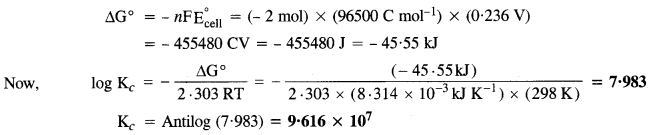Question 7.
Why does the conductivity of a solution decrease with dilution?
Conductivity of a solution is the conductance of ions present in a unit volume of the solutions. On dilution, no. of ions per unit volume decreases. Hence, the conductivity decreases.

Question 8.
Suggest a way to determine the $${ A }_{ m }^{ \circ }$$ for water.
Water (H2O) is a weak electrolyte. Its molar conductance at infinite dilution i.e., $${ A }_{ m }^{ \circ }$$ can be determined in terms of $${ A }_{ m }^{ \circ }$$ for strong electrolytes. This is in accordance with Kohlrausch’s Law.Question 9.
The molar conductance of 0·025 mol L-1of methanoic acid is 46·15 cm2 mol-1. Calculate its degree of dissociation and dissociation constant. Given λ°(H+) = 349·6 S cm2 mol-1 and λ°(HCOO) = 54·6 S cm2 mol-1.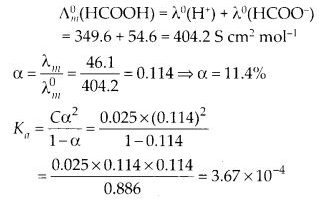Question 10.
If a current of 0·5 ampere flows through a metallic wire for 2 hours, then how many electrons flow through the wire ?
Quantity of charge (Q) passed = Current in amperes x Time in seconds = (0·5 A) X (2 x 60 x 60 s)
= 3600 As = 3600 C
No. of electrons flowing through the wire by passing a charge of one faraday (96500 C) = 6·022 x 1023
No. of electrons flowing through the wire by passing a charge of 3600 CQuestion 11.
Suggest a list of metals that are extracted electrolytically.
Na, Ca, Mg and Al

Question 12.
Consider the reaction :
Cr2$${ O }_{ 7 }^{ 2- }$$ + 14H+ + 6e → 2Cr3+ + 7H2O.
What is the quantity of electricity in coulombs needed to reduce 1 mole of Cr2$${ O }_{ 7 }^{ 2- }$$ ions ?
The quantity of electricity in coulombs is 6 F or 6 x 96500 C = 5·76 x 105 C.

Question 13.
Write the chemistry of recharging the lead storage battery highlighting all the materials that are involved during recharging.
Chemical reactions while recharging :
Chemical reactions while recharging :
2PbSO4 + 2H2O → PbO2 + Pb + 2H2SO4
Electricity is passed through the electrolyte PbSO4 which is converted into PbO2 and Pb.
Recharging is possible in this case because the PbSO4 formed during discharging is a solid and sticks to the electrodes. Therefore, it can either take up or give electrons during recharging.

Question 14.
Suggest two materials other than hydrogen that can be used as fuels in fuel cells.
Methane and Methanol.

Question 15.
Explain how rusting of iron can be envisaged as the setting up of an electrochemical cell.
Iron (Fe) is involved in the redox-reaction that is carried in the electrochemical cell which is set up. As a result, it slowly dissolves and the metal surface gets rusted or corroded.
The redox-reaction may be described as follows :
At anode: Fe (s) undergoes oxidation to release electrons
F2(s) → Fe2+(aq) + 2e–              ….(oxidation)
At cathode: The electrons which are released participate in the reduction reaction and combine with H+ ions released from carbonic acid (H2CO3) formed by the combination of CO2 and H2O present.NCERT EXERCISE

Question 1.
Arrange the following metals in the order in which they displace each other from the solution of their salts: Al, Cu, Fe, Mg and Zn.
Mg, Al, Zn, Fe, Cu, Ag.

Question 2.
Given the standard electrode potentials
K+/K = – 2·93 V, Ag+/Ag = 0·80 V
Hg2+/Hg = 0·79 V ; Mg2+/Mg = – 2·37V, Cr3+/Cr = – 0·74 V
Arrange these metals in increasing order of their reducing power.
Less the electrode potential more will be the reducing power.
Ag < Hg < Cr < Mg < K.

Question 3.
Depict the galvanic cell in which the reaction
Zn(s) + 2Ag+(aq) → Zn2+(aq) + 2Ag(s) takes place. Further show :
(i) which electrode is negatively charged ?
(ii) the carriers of the current in the cell.
(iii) individual reaction at each electrode. (C.B.S.E. Delhi 2008)
The galvanic cell in which the given reaction takes place is depicted as:
Zn(s) | Zn2+ (aq) || Ag+ (aq) | Ag(s)
(i) Zn electrode (anode) is negatively charged
(ii) Tons are carriers of current in the cell and in the external circuit, current from silver to Zinc.
(iii) The reaction taking place at the anode is given by,
Zn(s) -H → Zn2+(aq) + 2e
The reaction taking place at the cathode is given
Ag++ e → Ag(s)

Question 4.
Calculate the standard cell potentials of galvanic cell in which the following reactions take place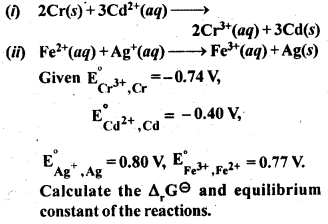Question 5.
Write the Nernst Equation and calculate e.m.f. of the following cells at 298 K :
(i) Mg(s) | Mg2+ (0·001 M) || Cu2+ (0·0001 M) | Cu(s) (C.B.S.E. Delhi 2008, 2013)
(ii) Fe(s) | Fe2+ (0·001 M) || H+ (1 M) | H2(g) (1 bar) | Pt(s)
(iii) Sn(s) | Sn2+ (0·050 M) || H+ (0·02 M) | H2(g) (1 bar) | Pt(s) (C.B.S.E. Outside Delhi 2013, 2015)
(iv) Pt(s) | Br2(l) | Br (0·010 M) || H+ (0·030 M) | H2(g) (1 bar) | Pt(s)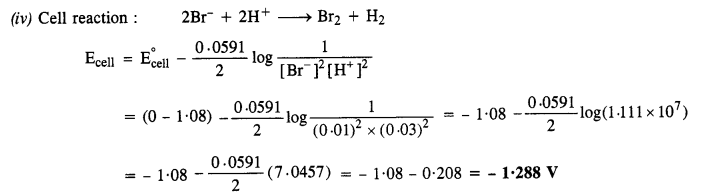Question 6.
In the button cell widely used in watches and in other devices, the following reaction takes place:
Zn (s) + Ag2O (s) + H2O (l) → Zn2+ (aq) + 2Ag (s) + 2OH (aq)
Determine E° and ∆G° for the reaction. (C.B.S.B. Delhi 2005, Outside Delhi 2006 Supp., 2008, C.B.S.E. Sample Paper 2010)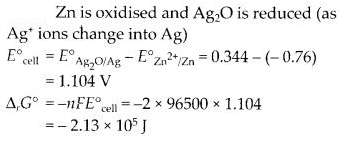Question 7.
Define conductivity and molar conductivity for the solution of an electrolyte. Discuss their variation with concentration.
The conductivity of a solution is defined as the conductance of a solution 1 cm in length and the area of cross-section cm2.1 is represented by K.

Conductivity always decreases with a decrease in concentration both for weak and strong electrolytes. This is because the number of ions per unit volume that carry the current in a solution decreases with a decrease in concentration.

Molar conductivity of a solution at a given concentration is the conductance of volume V of a solution containing 1 mole of the electrolyte kept between two electrodes with the area of cross-section A and distance of unit length.

Molar conductivity increases with a decrease in concentration. This is because the total volume of the solution containing one mole of the electrolyte increases on dilution.

Question 8.
The conductivity of 0·20 M solution of KCl at 298 K is 0·0248 S cm-1. Calculate its molar conductivity.
(C.B.S.E. Delhi 2008, 2013)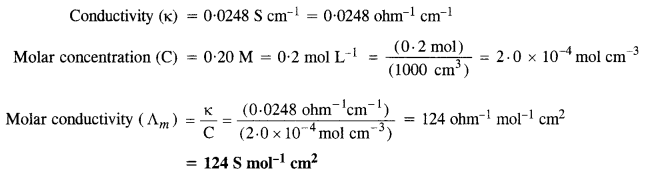Question 9.
The resistance of a conductivity cell containing 0·001 M KCl solution at 298 K is 1500 Ω. What is the cell constant if the conductivity of 0·001M KCl solution at 298 K is 0·146 x 10-3 S cm-2? (C.B.S.E. Outside Delhi 2007, 2008, 2013)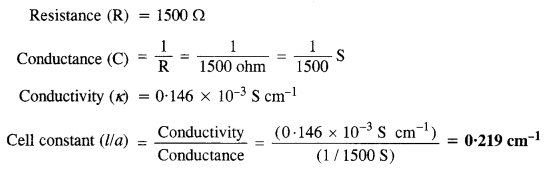Question 10.
The conductivity of sodium chloride solution at 298 K has been determined at different concentrations and results are given below :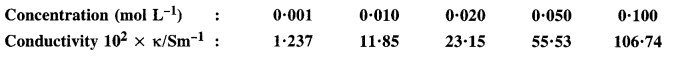Calculate molar conductivity for all the concentrations and draw a plot between $${ A }_{ m }^{ c }$$ and $$\sqrt { c }$$. Find the value $${ A }_{ m }^{ \circ }$$ from the graph.
$$\frac { 1S{ cm }^{ -1 } }{ 100S{ m }^{ -1 } } =1$$ (unit conversion factor)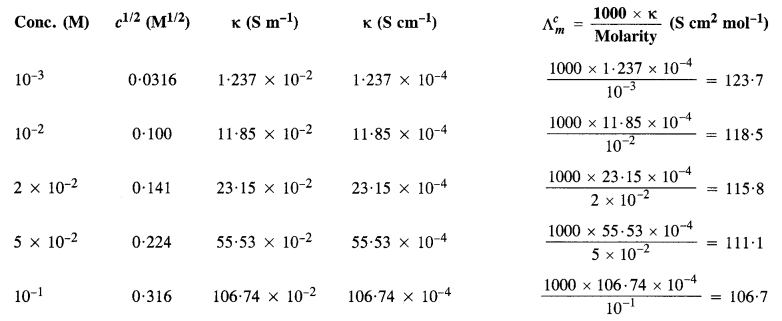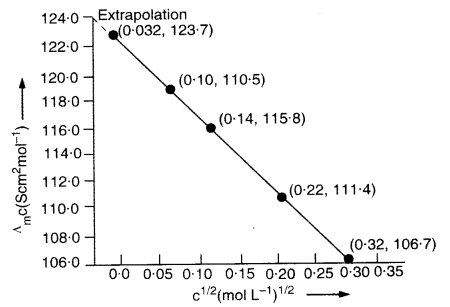$${ A }_{ m }^{ \circ }$$ can be obtained on extrapolation to zero concentration along Y-axis. It is 124·0Scm2mol-1.

Question 11.
The conductivity of 0·00241 M acetic acid is 7·896 x 10-5 S cm-1. Calculate the molar conductivity. If A° for acetic acid is 390·5 S cm2 mol-1, what is its dissociation constant? (C.B.S.E. Delhi 2008, C.B.S.E. Outside Delhi 2016)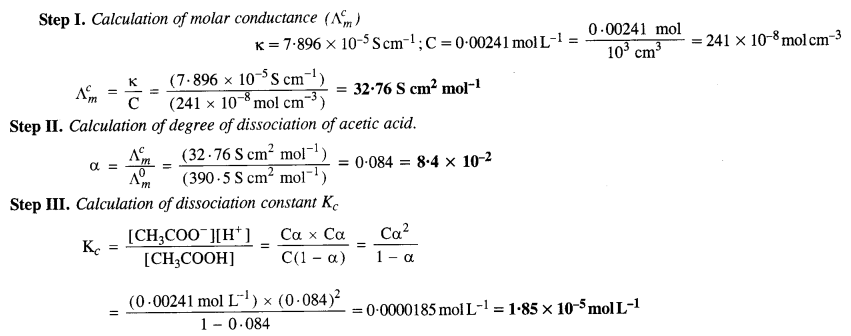Question 12.
How much charge is required for the reduction of :
(i) 1 mol of Al3+ to Al
(ii) 1 mol of Cu2+ to Cu
(iii) 1 mol of Mn$${ O }_{ 4 }^{ – }$$ to Mn2+.Question 13.
How much electricity in terms of Faraday is required to produce.
(i) 20.0 g of Ca from molten CaCl2?
(ii) 40.0 g of Al from molten Al203?Question 14.
How much electricity is required in coulomb for the oxidation of
(i) 1 mol of H2O to O2
(ii) 1 mol of FeO to Fe2O3.For the oxidation of two moles of FeO, charge required = 2 F
For the oxidation of one mole of FeO, charge required = 1 F = 96500 C.

Question 15.
A solution of Ni(NO3)2 is electrolyzed between platinum electrodes using a current of 5·0 ampere for 20 minutes. What weight of Ni will be produced at the cathode? (Atomic mass of Ni = 58·7). (Jharkhand Board 2009)Question 16.
Three electrolytic cells A, B, and C containing electrolytes zinc sulphate, silver nitrate, and copper sulphate respectively, were connected in series. A steady current of 1·50 ampere was passed through them until 1·45 g of silver was deposited at the cathode of cell B. How long did the current flow? What weight of copper and of zinc were deposited? (Atomic mass of Cu = 63·5 ; Zn = 65·3; Ag = 108) (C.B.S.E. Outside Delhi 2008, Jharkhand Board 2010)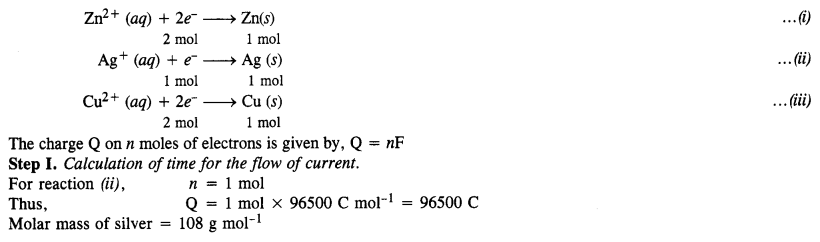Question 17.
Predict if the reaction between the following is feasible: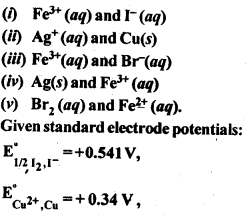The reaction is feasible if the EMF of the cell reaction is positive.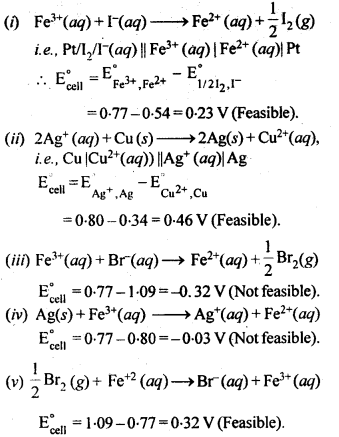Question 18.
Predict the products of electrolysis of each of the following :
(i) An aqueous solution of AgNO3 using silver electrodes.
(ii) An aqueous solution of AgNO3 using platinum electrodes.
(iii) A dilute solution of H2SO4 using platinum electrodes. (C.B.S.E. Outside Delhi 2007)
(iv) An aqueous solution of CuCl2 using platinum electrodes. (C. B. S. E. Sample Paper 2010)
(i) An aqueous solution of AgNO3 using silver electrodes :
Both AgNO3 and water will ionise in aqueous solutionAt cathode: Ag+ ions with less discharge potential are reduced in preference to H+ ions which will remain in solution. As a result, silver will be deposited at cathode.
Ag+ (aq) + e → Ag (deposited)
At anode: An equivalent amount of silver will be oxidised to Ag+ ions by releasing electrons.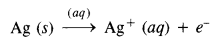(ii) An aqueous solution of AgNO3 using platinum electrodes:

In this case, the platinum electrodes are the non-attackable electrodes. On passing current the following changes will occur at the electrodes.
At cathode: Ag+ ions will be reduced to Ag which will get deposited at the cathode.
At anode: Both $${ NO }_{ 3 }^{ – }$$ and OH ions will migrate. But OH ions with less discharge potential will be oxidised in preference to $${ NO }_{ 3 }^{ – }$$ ions which will remain in solution.Thus, as a result of electrolysis, silver is deposited on the cathode while O2 is evolved at the anode. The solution will be acidic due to the presence of HNO3.
(iii) A dilute solution of H2SO4 using platinum electrodes:
On passing current, both acid and water will ionise as follows:At cathode:
H+ (aq) ions will migrate to the cathode and will be reduced to H2.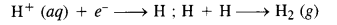Thus, H2 (g) will be evolved at the cathode.
At anode: OH ions will be released in preference to $${ SO }_{ 4 }^{ 2- }$$ ions because their discharge potential is less. They will be oxidized as follows: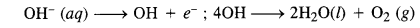Thus, O2 (g) will be evolved at the anode. The solution will be acidic and will contain H2SO4.
(iv) An aqueous solution of CuCl2 using platinum electrodes :
The electrolysis proceeds in the same manner as discussed in the case of AgNO3 solution. Both CuCl2 and H2O will ionise as follows :At cathode:
Cu2+ ions will be reduced in preference to H+ ions and copper will be deposited at the cathode

Cu2+ (aq) + 2e → Cu (deposited)

At anode: C ions will be discharged in preference to OH ions which will remain in solution.

Cl → Cl + e; Cl + Cl → Cl2 (g)

Thus, Cl2 will be evolved at the anode.

We hope the NCERT Solutions for Class 12 Chemistry Chapter 3 Electrochemistry help you. If you have any query regarding NCERT Solutions for Class 12 Chemistry Chapter 3 Electrochemistry, drop a comment below and we will get back to you at the earliest.